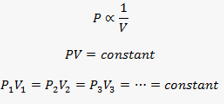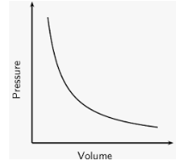# Boyles Law Formula

## Boyles Law Formulas and Examples

According to this experimental gas law, the pressure of a given mass of an ideal gas is inversely proportional to its volume at a constant temperature. An important point to note here is that the amount of ideal gas (i.e., number of moles) is kept fixed and a constant temperature is maintained. Under this condition, the pressure of the gas will tend to increase if we decrease the volume of the container and vice-versa.

Mathematically, it can be expressed as:Where ‘P’ is the pressure exerted by the gas and ‘V’ is the Volume of the container.

Graphically, we can observe the behaviour of the curve as follows:You can see how the value of Pressure decreases when the Volume is increased or vice-versa.

In order to understand it better, let us go through a simple example:

Example: If 20$c{m^3}$ gas at 1 atm. is expanded to 50 $c{m^3}$ at constant T, then what is the final pressure
Options:
(a) $20 \times \frac{1}{{50}}$
(b) $50 \times \frac{1}{{20}}$
(c) $1 \times \frac{1}{{20}} \times 50$
(d) None of these
Solution:
At constant $T,{P_1}{V_1} = {P_2}{V_2}$
$1 \times 20 = {P_2} \times 50$; ${P_2} = \frac{{20}}{{50}} \times 1$

We hope you have understood, how to apply the Boyle’s Law Formula. Now in order to test your understanding, try and solve the Question by yourself.

Example: An ideal gas exerts a pressure of 3atm in a 3L container. The container is at a temperature of 298K. What will be the final pressure if the volume of the container changes to 2L?
Options:
(a) 2 atm
(b) 3.5 atm
(c) 4.5 atm
(d) 4 atm
${P_1}{V_1} = {P_2}{V_2}$